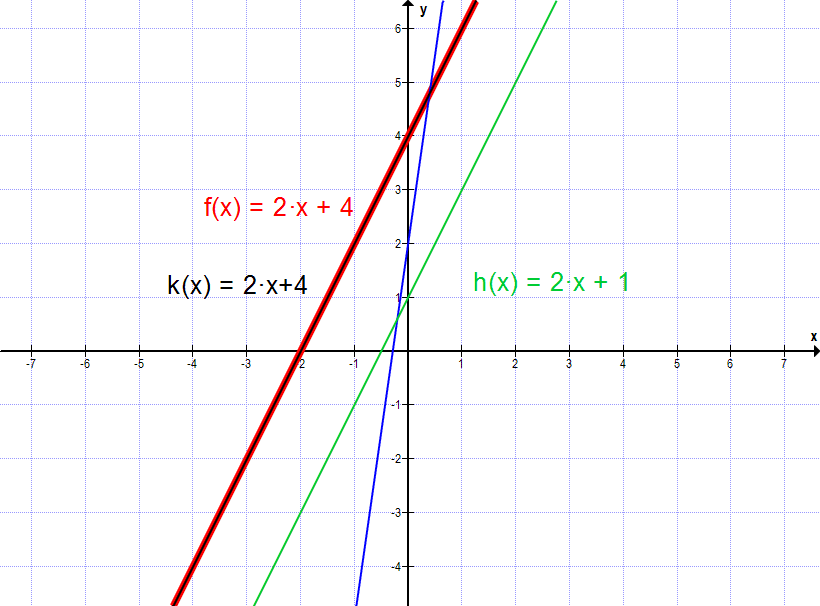# Can you cut graphs

## Intersection of Lines - Introduction

A straight line is a straight line that we draw in the coordinate system. It is described by a functional equation such as.

Two straight lines can intersect at one, zero or several points. We consider these cases below.

As is already known, the normal form of a linear function is given as:

If we now have two linear functions, we can investigate the position of both lines in relation to one another.

There are three cases:

1. Exactly one intersection
2. No intersection
3. Infinite number of intersections

Let's take a closer look at the cases.

### possible solutions

If we have two straight lines and are interested in their position in relation to one another, we have to equate the functional equations.

Equate means we have to write them side by side in the form of an equation:. If we solve the resulting equation, several cases can arise for the solution:

1. Exactly one solution comes out. → We have exactly one point of intersection.

2. The equation is untrue. No solution. → We don't have an intersection. The straight lines are parallel.

3. The equation is always true. An infinite number of solutions. → The straight lines lie on top of one another (they are identical).

### Illustration on the graph

The following figure shows all possible solutions with examples of function graphs.and have an intersection

and have no intersection

and have an infinite number of intersections.

Next, let's look at how we can computationally determine intersections.